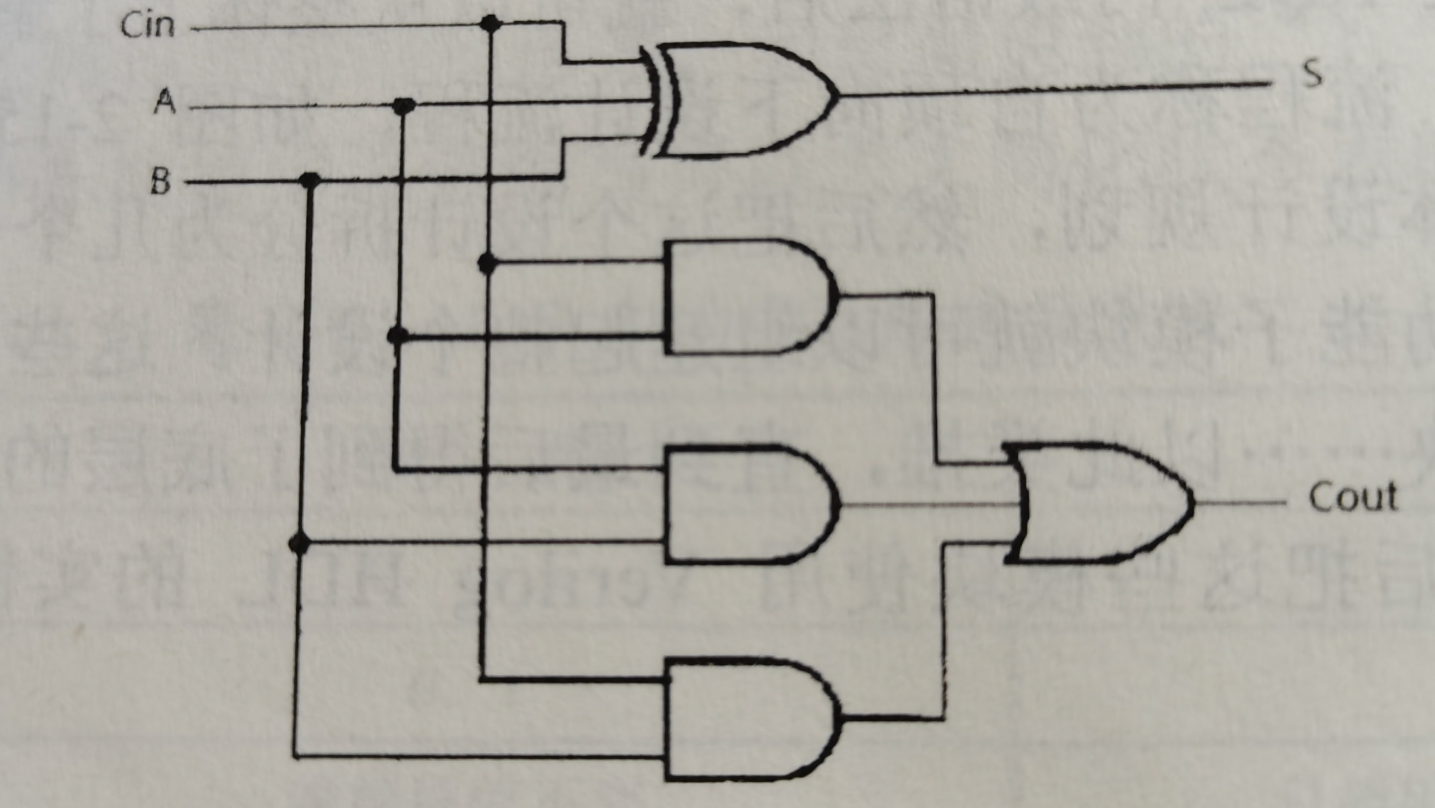# 4位全加器的门级建模

## 1位全加器门级描述过程

1位全加器的设计思路就是“翻译”电路图。电路图如下：### 代码

Verilog代码的编写要有两部分：设计代码和测试代码，门级建模中设计代码编写是最简单的，只需要会认电路图中的门是什么，再掌握Verilog编码规则就可以轻松的编写代码。有关测试代码的编写先简单说明一下，测试就是验证设计代码是否满足“预期的结果”。

### 1位全加器门级描述代码

module	add_1_m	(a,b,cin,cout,sum);
input	a,b;
input	cin;
output	cout;
output	sum;
wire	[2:0]	w;
and
a1(w,a,cin),
a2(w,a,b),
a3(w,b,cin);
xor	a4(sum,a,b,cin);
or	a5(cout,w,w,w);
endmodule


### 1位全加器测试代码

module	add_1_m_tb;
reg	a,b;
reg	cin;
wire	cout1;
wire	sum1;

initial
begin
#00	a=0;b=0;cin=0;
#10	a=0;b=1;
#10	a=1;b=0;
#10	a=1;b=1;
#00	a=0;b=0;cin=1;
#10	a=0;b=1;
#10	a=1;b=0;
#10	a=1;b=1;
end
initial
begin
$monitor($realtime,"\ta=%d\tb=%d\tcin=%d\t\t\tcout1=%d\tsum1=%d\t",a,b,cin,cout1,sum1);
end
endmodule### 4位全加器门级描述代码

//use add_1_m connect to add_4
input	[3:0]	a,b;
input		cin;
output		cout;
output	[3:0]	sum;
wire	[3:1]	c;
endmodule


### 4位全加器测试代码

module add_4_tb;
reg	[3:0]	a,b;
reg		cin;
wire		cout1;
wire	[3:0]	sum1;
integer seed1,seed2,seed3;
initial
begin
seed1=1;seed2=2;seed3=3;
a=0;b=0;cin=0;
repeat(10)
begin
#10	a=($random(seed1)/16);b=($random(seed2)/16);cin=($random(seed3)/2); end #10$stop;
end

initial	$monitor($realtime,"\ta=%d\tb=%d\tcin=%d\t\t\tcout1=%d\tsum1=%d",a[3:0],b[3:0],cin,cout1,sum1[3:0]);
endmodule## 感想

03-22
03-252
01-134216
10-191万+
01-05354
11-22
10-24258
08-01611
08-04650
05-128727
08-092480
09-09982
11-22
03-032万+
06-222134
01-142万+
04-221471
12-07769
07-201058

### “相关推荐”对你有帮助么？

•非常没帮助
•没帮助
•一般
•有帮助
•非常有帮助被折叠的  条评论 为什么被折叠?到【灌水乐园】发言¥2 ¥4 ¥6 ¥10 ¥20余额支付 (余额：-- )扫码支付获取中扫码支付点击重新获取扫码支付1.余额是钱包充值的虚拟货币，按照1:1的比例进行支付金额的抵扣。
2.余额无法直接购买下载，可以购买VIP、C币套餐、付费专栏及课程。余额充值